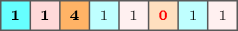## 5.320. period_except_0

Origin

Derived from $\mathrm{𝚙𝚎𝚛𝚒𝚘𝚍}$.

Constraint

$\mathrm{𝚙𝚎𝚛𝚒𝚘𝚍}_\mathrm{𝚎𝚡𝚌𝚎𝚙𝚝}_\mathtt{0}\left(\mathrm{𝙿𝙴𝚁𝙸𝙾𝙳},\mathrm{𝚅𝙰𝚁𝙸𝙰𝙱𝙻𝙴𝚂},\mathrm{𝙲𝚃𝚁}\right)$

Arguments
 $\mathrm{𝙿𝙴𝚁𝙸𝙾𝙳}$ $\mathrm{𝚍𝚟𝚊𝚛}$ $\mathrm{𝚅𝙰𝚁𝙸𝙰𝙱𝙻𝙴𝚂}$ $\mathrm{𝚌𝚘𝚕𝚕𝚎𝚌𝚝𝚒𝚘𝚗}\left(\mathrm{𝚟𝚊𝚛}-\mathrm{𝚍𝚟𝚊𝚛}\right)$ $\mathrm{𝙲𝚃𝚁}$ $\mathrm{𝚊𝚝𝚘𝚖}$
Restrictions
 $\mathrm{𝙿𝙴𝚁𝙸𝙾𝙳}\ge 1$ $\mathrm{𝙿𝙴𝚁𝙸𝙾𝙳}\le |\mathrm{𝚅𝙰𝚁𝙸𝙰𝙱𝙻𝙴𝚂}|$ $\mathrm{𝚛𝚎𝚚𝚞𝚒𝚛𝚎𝚍}$$\left(\mathrm{𝚅𝙰𝚁𝙸𝙰𝙱𝙻𝙴𝚂},\mathrm{𝚟𝚊𝚛}\right)$ $\mathrm{𝙲𝚃𝚁}\in \left[=,\ne ,<,\ge ,>,\le \right]$
Purpose

Let us note ${V}_{0},{V}_{1},\cdots ,{V}_{m-1}$ the variables of the $\mathrm{𝚅𝙰𝚁𝙸𝙰𝙱𝙻𝙴𝚂}$ collection. $\mathrm{𝙿𝙴𝚁𝙸𝙾𝙳}$ is the period of the sequence ${V}_{0}{V}_{1}\cdots {V}_{m-1}$ according to constraint $\mathrm{𝙲𝚃𝚁}$. This means that $\mathrm{𝙿𝙴𝚁𝙸𝙾𝙳}$ is the smallest natural number such that ${V}_{i}\mathrm{𝙲𝚃𝚁}{V}_{i+\mathrm{𝙿𝙴𝚁𝙸𝙾𝙳}}\vee {V}_{i}=0\vee {V}_{i+\mathrm{𝙿𝙴𝚁𝙸𝙾𝙳}}=0$ holds for all $i\in 0,1,\cdots ,m-\mathrm{𝙿𝙴𝚁𝙸𝙾𝙳}-1$.

Example
$\left(3,〈1,1,4,1,1,0,1,1〉,=\right)$

The $\mathrm{𝚙𝚎𝚛𝚒𝚘𝚍}_\mathrm{𝚎𝚡𝚌𝚎𝚙𝚝}_\mathtt{0}$ constraint holds since, as depicted by Figure 5.320.1, its first argument $\mathrm{𝙿𝙴𝚁𝙸𝙾𝙳}=3$ is equal (i.e., since $\mathrm{𝙲𝚃𝚁}$ is set to $=$) to the period of the sequence $11411011$; value 0 is assumed to be equal to any other value.

##### Figure 5.320.1. A sequence that has a period of 3 when we assume that value 0 can match to any other valueTypical
 $\mathrm{𝙿𝙴𝚁𝙸𝙾𝙳}>1$ $\mathrm{𝙿𝙴𝚁𝙸𝙾𝙳}<|\mathrm{𝚅𝙰𝚁𝙸𝙰𝙱𝙻𝙴𝚂}|$ $|\mathrm{𝚅𝙰𝚁𝙸𝙰𝙱𝙻𝙴𝚂}|>2$ $\mathrm{𝚛𝚊𝚗𝚐𝚎}$$\left(\mathrm{𝚅𝙰𝚁𝙸𝙰𝙱𝙻𝙴𝚂}.\mathrm{𝚟𝚊𝚛}\right)>1$ $\mathrm{𝚊𝚝𝚕𝚎𝚊𝚜𝚝}$$\left(1,\mathrm{𝚅𝙰𝚁𝙸𝙰𝙱𝙻𝙴𝚂},0\right)$ $\mathrm{𝙲𝚃𝚁}\in \left[=\right]$
Symmetries
• Items of $\mathrm{𝚅𝙰𝚁𝙸𝙰𝙱𝙻𝙴𝚂}$ can be reversed.

• Items of $\mathrm{𝚅𝙰𝚁𝙸𝙰𝙱𝙻𝙴𝚂}$ can be shifted.

• All occurrences of two distinct values of $\mathrm{𝚅𝙰𝚁𝙸𝙰𝙱𝙻𝙴𝚂}.\mathrm{𝚟𝚊𝚛}$ that are both different from 0 can be swapped; all occurrences of a value of $\mathrm{𝚅𝙰𝚁𝙸𝙰𝙱𝙻𝙴𝚂}.\mathrm{𝚟𝚊𝚛}$ that is different from 0 can be renamed to any unused value that is also different from 0.

Arg. properties
• Functional dependency: $\mathrm{𝙿𝙴𝚁𝙸𝙾𝙳}$ determined by $\mathrm{𝚅𝙰𝚁𝙸𝙰𝙱𝙻𝙴𝚂}$ and $\mathrm{𝙲𝚃𝚁}$.

• Contractible wrt. $\mathrm{𝚅𝙰𝚁𝙸𝙰𝙱𝙻𝙴𝚂}$ when $\mathrm{𝙲𝚃𝚁}\in \left[=\right]$ and $\mathrm{𝙿𝙴𝚁𝙸𝙾𝙳}=1$.

• Prefix-contractible wrt. $\mathrm{𝚅𝙰𝚁𝙸𝙰𝙱𝙻𝙴𝚂}$.

• Suffix-contractible wrt. $\mathrm{𝚅𝙰𝚁𝙸𝙰𝙱𝙻𝙴𝚂}$.

Usage

Useful for timetabling problems where a person should repeat some work pattern over an over except when he is unavailable for some reason. The value 0 represents the fact that he is unavailable, while the other values are used in the work pattern.

Algorithm